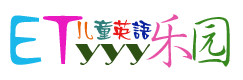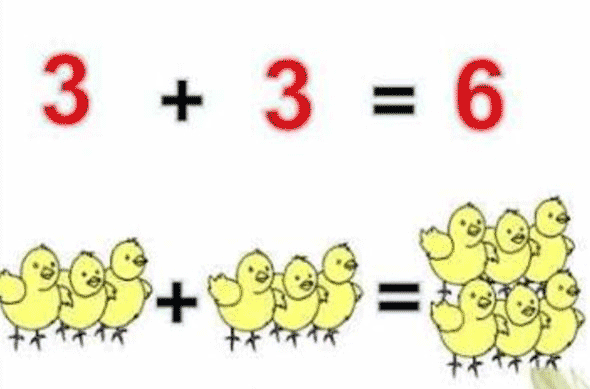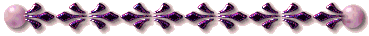学字母 | 学单词 |学美语 | 音标 | 会话 | 听歌学英语 | 卡通动画 | 精选推荐 | 网上采集 | 儿童酷站跟我学美语第一册Say it out loud.

1+2=2 One plus one equals two.
2+2=4 Two plus two equals four.
3+3=6 Three plus three equals six.

Let's guess.
1+?=3 One plus what equals three?
I know, I know.
O.K. Johnny?
Johnny: 2, 1+2=3
Very good!

2+?=9 Two plus what equals nine?
I know, I know.
O.K. Amy?
Amy: 7, 2+7=9
Techer: Very good!